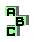EXTANGENTIAL TRIANGLE

 r50 tangential triangle, intangential triangle, homothetic triangles, point X(55) d Clawson point r410 excircles, incircle, homothetic triangles r570 perspective, point X(65) r692 Yff central triangle r1801 centroid, incenter, excenters, circumcenter, parallelogic triangles